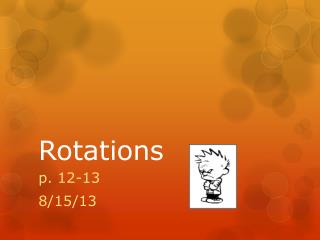# Rotations - PowerPoint PPT PresentationDownload PresentationRotations

RotationsDownload Presentation## Rotations

- - - - - - - - - - - - - - - - - - - - - - - - - - - E N D - - - - - - - - - - - - - - - - - - - - - - - - - - -
##### Presentation Transcript

1. Rotations p. 12-13 8/15/13

2. Review- Left Side p. 12 1) Using point (1,2), complete the following transformation ( x, y )→( x - 2, y + 1 ) 2) Reflect A(-9, -3) over the y-axis. 3) Reflect B(-7, 0) over the y-axis.

3. Rotations p. 13 • Objective: Rotate figures in the coordinate plane.

4. Rotations • A rotation is a 1)rigid transformation in which a 2)figure is rotated, or turned, about a fixed point. The3)center of rotation is the fixed point (0,0). A rotation does not change the size or shape of the figure.

5. Examples of Rotations

6. Examples of Rotations

7. Examples of Rotations

8. Rotations are described using degrees and directions • Degree: 90ᵒ, 180ᵒ, 270ᵒ • Direction: Clockwise or Counterclockwise

9. What happens when we rotate the rectangle 90 degrees?

10. Rules Clockwise Counterclockwise

11. Left Side Practice • Use the point (-2,3) 1. Rotate counterclockwise 90 degrees 2. Rotate clockwise 180 degrees 3. Rotate counterclockwise 270 degrees • A triangle has vertices of A (0,0); B (3,0); and C (3,4) 4. Rotate the triangle 90 degrees counterclockwise about the origin. Name the the coordinates of the vertices using A‘, B‘, and C'